# Histogram stretching

One of the other advantage of Histogram s that we discussed in our tutorial of introduction to histograms is contrast enhancement.

There are two methods of enhancing contrast. The first one is called Histogram stretching that increase contrast. The second one is called Histogram equalization that enhance contrast and it has been discussed in our tutorial of histogram equalization.

Before we will discuss the histogram stretching to increase contrast , we will briefly define contrast.

## Contrast.

Contrast is the difference between maximum and minimum pixel intensity.

Consider this image.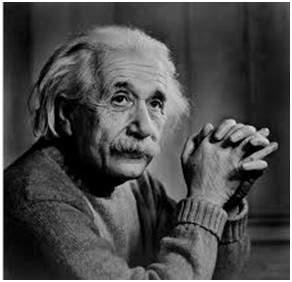The histogram of this image is shown below.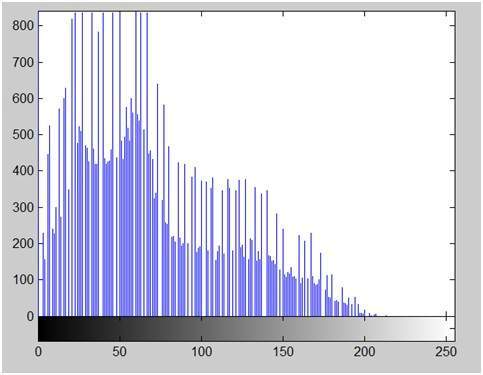Now we calculate contrast from this image.

Contrast = 225.

Now we will increase the contrast of the image.

## Increasing the contrast of the image:

The formula for stretching the histogram of the image to increase the contrast is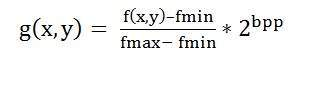The formula requires finding the minimum and maximum pixel intensity multiply by levels of gray. In our case the image is 8bpp, so levels of gray are 256.

The minimum value is 0 and the maximum value is 225. So the formula in our case is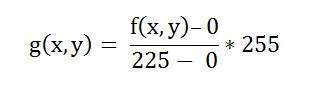where f(x,y) denotes the value of each pixel intensity. For each f(x,y) in an image , we will calculate this formula.

After doing this, we will be able to enhance our contrast.

The following image appear after applying histogram stretching.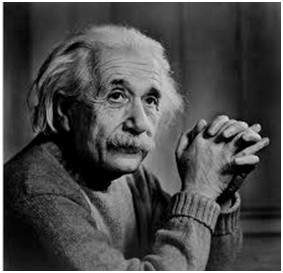The stretched histogram of this image has been shown below.

Note the shape and symmetry of histogram. The histogram is now stretched or in other means expand. Have a look at it.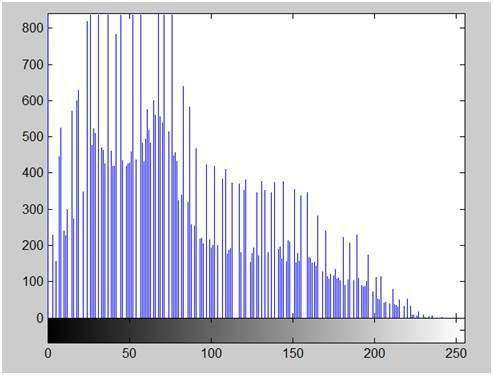In this case the contrast of the image can be calculated as

Contrast = 240

Hence we can say that the contrast of the image is increased.

Note: this method of increasing contrast doesnot work always, but it fails on some cases.

## Failing of histogram stretching

As we have discussed , that the algorithm fails on some cases. Those cases include images with when there is pixel intensity 0 and 255 are present in the image

Because when pixel intensities 0 and 255 are present in an image , then in that case they become the minimum and maximum pixel intensity which ruins the formula like this.

Original FormulaPutting fail case values in the formula: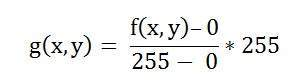Simplify that expression gives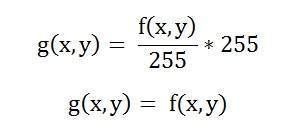That means the output image is equal to the processed image. That means there is no effect of histogram stretching has been done at this image.